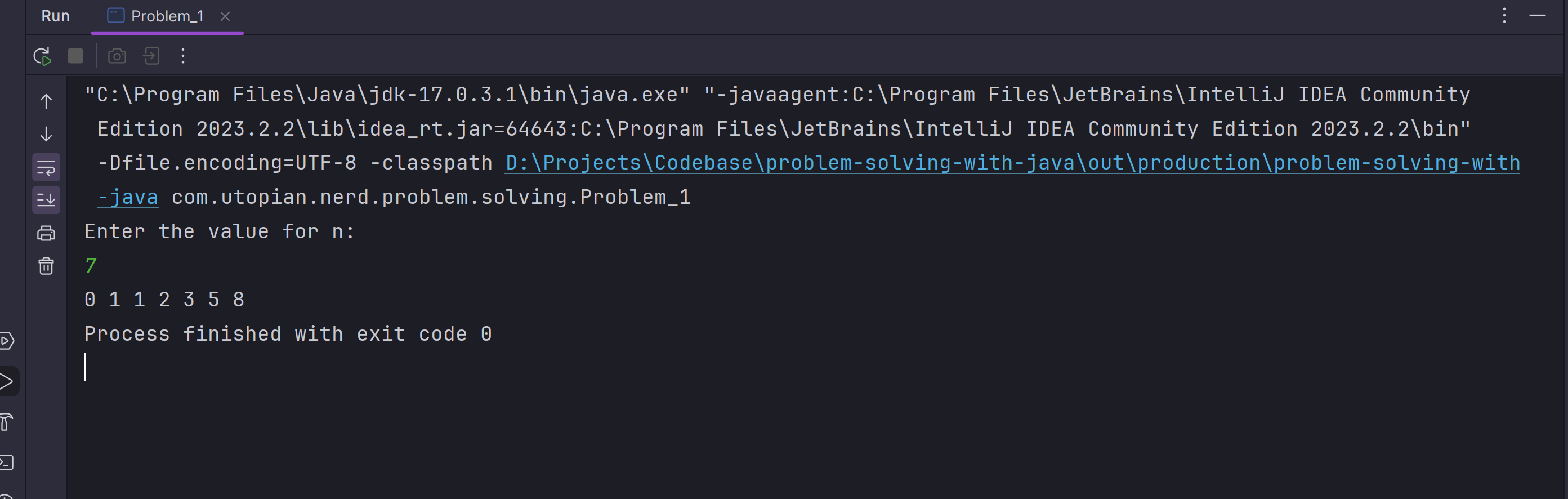## 🎯 Overview#

Fibonacci Numbers are series of numbers: `0, 1, 1, 2, 3, 5, 8, 13, 21, 34, ...`

The first fibonacci number in the series is: `0`.

The second fibonacci number in the series is: `1`.

From the third number and onwards in the series, we can get that number by adding the previous two fibonacci numbers. So the third fibonacci number will be: `0 + 1 = 1`.

The fourth fibonacci number in the series will be: `1 + 1 = 2`.

The fifth fibonacci number in the series will be: `1 + 2 = 3`. . . . and so on.

## 🎯 Solving the problem in Java#

Problem Statement: Write a Java program to print first ‘n’ Fibonacci Numbers.

``````/**
* Problem 1: Write a Java program to print first ‘n’ Fibonacci Numbers.
*/
public class Problem_1 {

public static void main(String[] args) {

System.out.println("Enter the value for n:");
Scanner sc = new Scanner(System.in);

int n = sc.nextInt();

int firstNumber = 0;
int secondNumber = 1;
int currentNumber;

for (int i = 0; i < n; i++) {
System.out.print(firstNumber + " ");
currentNumber = firstNumber + secondNumber;
firstNumber = secondNumber;
secondNumber = currentNumber;
}
}
}
``````

Output:## 🎯 Github#

Code shared in this post can be found here.